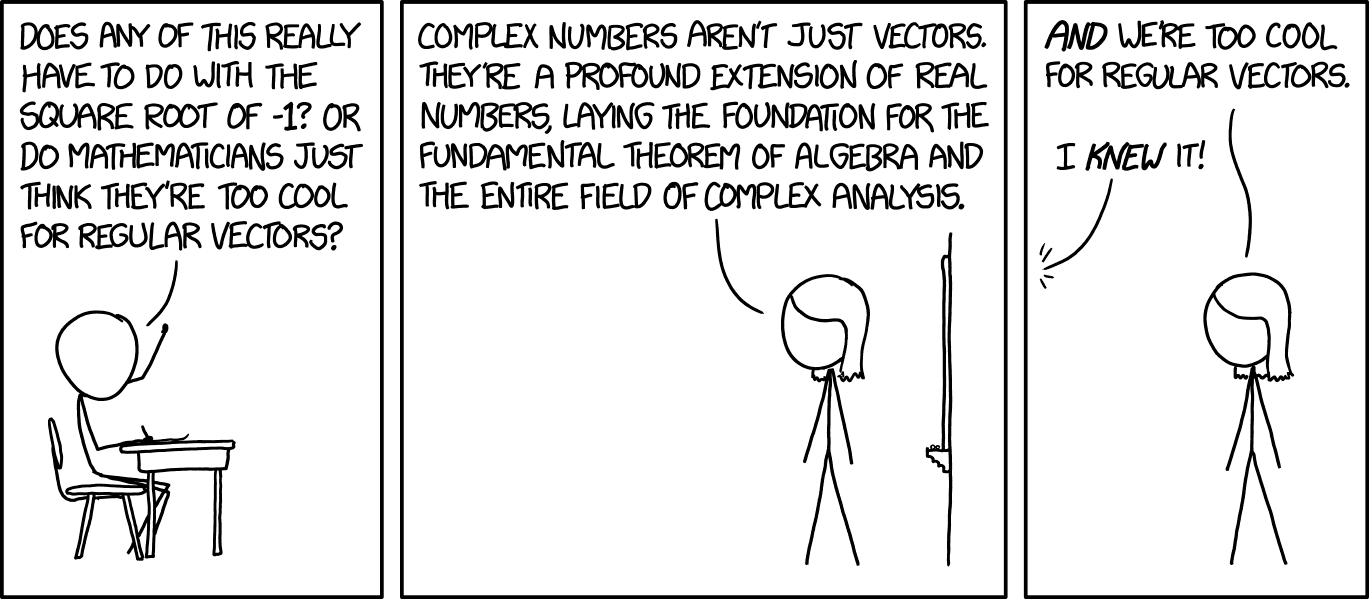Classes of Abed Nego Ragil Putra > PHP Inventory Management System with Scanner > vendor/markbaker/complex/README.md > Download
File: vendor/markbaker/complex/README.md Documentation text/markdown Documentation PHP Inventory Management System with Scanner Manage inventory stock using scanner application By Abed Nego Ragil Putra 2 years ago 4,542 bytes

## ContentsDownload

# PHPComplex

PHP Class for handling Complex numbersThe library currently provides the following operations:

- addition - subtraction - multiplication - division

``````- division by
- division into
``````

together with functions for

- theta (polar theta angle) - rho (polar distance/radius) - conjugate * negative - inverse (1 / complex) - cos (cosine) - acos (inverse cosine) - cosh (hyperbolic cosine) - acosh (inverse hyperbolic cosine) - sin (sine) - asin (inverse sine) - sinh (hyperbolic sine) - asinh (inverse hyperbolic sine) - sec (secant) - asec (inverse secant) - sech (hyperbolic secant) - asech (inverse hyperbolic secant) - csc (cosecant) - acsc (inverse cosecant) - csch (hyperbolic secant) - acsch (inverse hyperbolic secant) - tan (tangent) - atan (inverse tangent) - tanh (hyperbolic tangent) - atanh (inverse hyperbolic tangent) - cot (cotangent) - acot (inverse cotangent) - coth (hyperbolic cotangent) - acoth (inverse hyperbolic cotangent) - sqrt (square root) - exp (exponential) - ln (natural log) - log10 (base-10 log) - log2 (base-2 log) - pow (raised to the power of a real number)

# Usage

To create a new complex object, you can provide either the real, imaginary and suffix parts as individual values, or as an array of values passed passed to the constructor; or a string representing the value. e.g

``````\$real = 1.23;
\$imaginary = -4.56;
\$suffix = 'i';

\$complexObject = new Complex\Complex(\$real, \$imaginary, \$suffix);
``````

or

``````\$real = 1.23;
\$imaginary = -4.56;
\$suffix = 'i';

\$arguments = [\$real, \$imaginary, \$suffix];

\$complexObject = new Complex\Complex(\$arguments);
``````

or

``````\$complexString = '1.23-4.56i';

\$complexObject = new Complex\Complex(\$complexString);
``````

Complex objects are immutable: whenever you call a method or pass a complex value to a function that returns a complex value, a new Complex object will be returned, and the original will remain unchanged. This also allows you to chain multiple methods as you would for a fluent interface (as long as they are methods that will return a Complex result).

## Performing Mathematical Operations

To perform mathematical operations with Complex values, you can call the appropriate method against a complex value, passing other values as arguments

``````\$complexString1 = '1.23-4.56i';
\$complexString2 = '2.34+5.67i';

\$complexObject = new Complex\Complex(\$complexString1);
``````

or pass all values to the appropriate function

``````\$complexString1 = '1.23-4.56i';
\$complexString2 = '2.34+5.67i';

``````

If you want to perform the same operation against multiple values (e.g. to add three or more complex numbers), then you can pass multiple arguments to any of the operations.

You can pass these arguments as Complex objects, or as an array or string that will parse to a complex object.

## Using functions

When calling any of the available functions for a complex value, you can either call the relevant method for the Complex object

``````\$complexString = '1.23-4.56i';

\$complexObject = new Complex\Complex(\$complexString);
echo \$complexObject->sinh();
``````

or you can call the function as you would in procedural code, passing the Complex object as an argument

``````\$complexString = '1.23-4.56i';

\$complexObject = new Complex\Complex(\$complexString);
echo Complex\sinh(\$complexObject);
``````

When called procedurally using the function, you can pass in the argument as a Complex object, or as an array or string that will parse to a complex object.

``````\$complexString = '1.23-4.56i';

echo Complex\sinh(\$complexString);
``````

In the case of the `pow()` function (the only implemented function that requires an additional argument) you need to pass both arguments when calling the function procedurally

``````\$complexString = '1.23-4.56i';

\$complexObject = new Complex\Complex(\$complexString);
echo Complex\pow(\$complexObject, 2);
``````

or pass the additional argument when calling the method

``````\$complexString = '1.23-4.56i';

\$complexObject = new Complex\Complex(\$complexString);
echo \$complexObject->pow(2);
``````Advertise on this siteSite mapNewsletterStatisticsSite tipsPrivacy policyContact
For more information send a message to `info at phpclasses dot org`.